Next: Capacitance Up: Electric Potential Previous: Example 5.3: Electric potential due

## Example 5.4: Electric potential due to point charges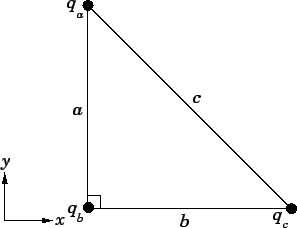Question: Suppose that three point charges,,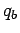, and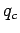, are arranged at the vertices of a right-angled triangle, as shown in the diagram. What is the absolute electric potential of the third charge if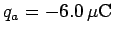,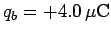,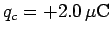,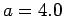m, and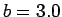m? Suppose that the third charge, which is initially at rest, is repelled to infinity by the combined electric field of the other two charges, which are held fixed. What is the final kinetic energy of the third charge?

Solution: The absolute electric potential of the third charge due to the presence of the first charge is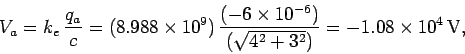where use has been made of the Pythagorean theorem. Likewise, the absolute electric potential of the third charge due to the presence of the second charge is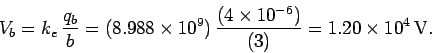The net absolute potential of the third charge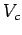is simply the algebraic sum of the potentials due to the other two charges taken in isolation. Thus,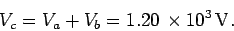The change in electric potential energy of the third charge as it moves from its initial position to infinity is the product of the third charge,, and the difference in electric potential (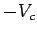) between infinity and the initial position. It follows that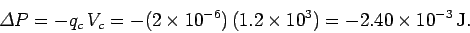This decrease in the potential energy of the charge is offset by a corresponding increase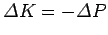in its kinetic energy. Since the initial kinetic energy of the third charge is zero (because it is initially at rest), the final kinetic energy is simply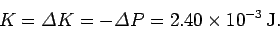Next: Capacitance Up: Electric Potential Previous: Example 5.3: Electric potential due
Richard Fitzpatrick 2007-07-14